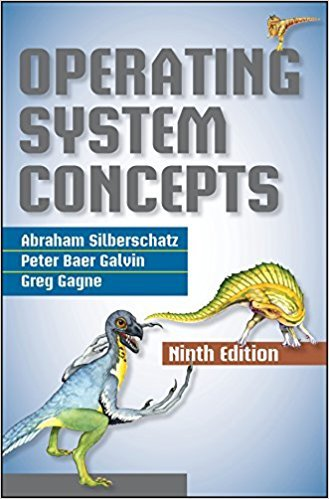×
×

# Many CPU-scheduling algorithms are parameterized. For example, theRR algorithm requiresISBN: 9781118063330 327

## Solution for problem 6.5 Chapter 6

Operating System Concepts | 9th Edition

• Textbook Solutions
• 2901 Step-by-step solutions solved by professors and subject experts
• Get 24/7 help from StudySoup virtual teaching assistantsOperating System Concepts | 9th Edition

4 5 1 387 Reviews
23
0
Problem 6.5

Many CPU-scheduling algorithms are parameterized. For example, theRR algorithm requires a parameter to indicate the time slice. Multilevelfeedback queues require parameters to define the number of queues, thescheduling algorithm for each queue, the criteria used to move processesbetween queues, and so on.These algorithms are thus really sets of algorithms (for example, theset of RR algorithms for all time slices, and so on). One set of algorithmsmay include another (for example, the FCFS algorithm is the RR algorithmwith an infinite time quantum). What (if any) relation holds between thefollowing pairs of algorithm sets?a. Priority and SJFb. Multilevel feedback queues and FCFSc. Priority and FCFSd. RR and SJF

Step-by-Step Solution:
Step 1 of 3

Key: Light Blue = main idea Purple = important parts of the main idea Green = examples Orange = key terms Pink = the definition of the key term Red = especially important Chapter 7: Deriving Energy from Food  Breathing and oxygen are a part of energy transfer  They are a part of a system that allows us to extract the energy that is used to put ATP together food...

Step 2 of 3

Step 3 of 3

##### ISBN: 9781118063330

Unlock Textbook Solution